# Geometry

## Objective

Use equations to solve for unknown angles. (Part 2)

## Common Core Standards

### Core Standards

?

• 7.G.B.5 — Use facts about supplementary, complementary, vertical, and adjacent angles in a multi-step problem to write and solve simple equations for an unknown angle in a figure.

?

• 4.MD.C.5

• 4.MD.C.7

## Criteria for Success

?

1. Identify angle relationships in angle diagrams involving vertical, supplementary, and complementary angles.
2. Write equations to represent relationships between known and unknown angle measurements.
3. Determine the measures of unknown angles and judge the reasonableness of the measures.

## Tips for Teachers

?

• Lessons 3 and 4 present students with more complicated line and angle diagrams where they use various relationships between the angles to set up equations and solve for unknown angle measurements. In Lesson 4, students encounter angles that are described by variables with a coefficient other than 1. They will distinguish between solving for the value of the variable and determining what the measure of the angle is.
• As with Lesson 3, some students may struggle with identifying the relevant relationships and setting up a corresponding equation; others may struggle with solving the equations. Keep an eye out for both/either struggles in order to address incorrect answers appropriately.
• Continue to have students describe what they see in the angle diagrams before they solve. This can support them in understanding the structure of the diagram (identifying key lines or features such as right angles) and in making sense of the diagram by identifying relationships between lines and angles (MP.7 and MP.8).

#### Fishtank Plus

• Problem Set
• Student Handout Editor
• Vocabulary Package

## Anchor Problems

?

### Problem 1

For each diagram below, write and solve an equation to determine the value of the variable. Then determine the measure of each angle in the diagrams.

1.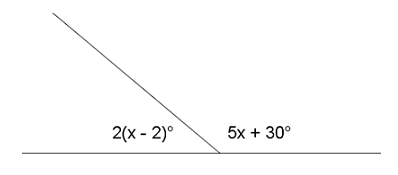1.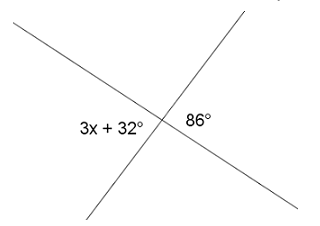1.

​​​​​​​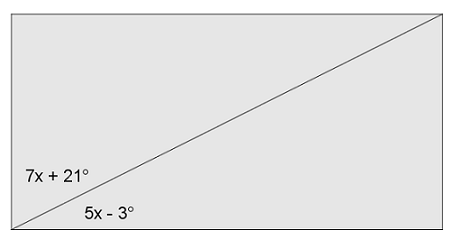### Problem 2

Two lines meet at a point that is also the endpoint of two rays.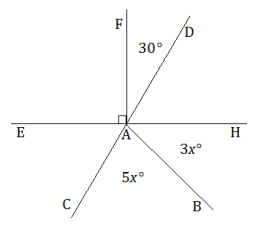1. Describe the angle relationships you see in the diagram.
2. Set up and solve an equation to find the value of ${x.}$
3. Find the measurements of ${\angle BAC}$ and ${\angle BAH}$.

#### References

EngageNY Mathematics Grade 7 Mathematics > Module 6 > Topic A > Lesson 3Example 3

Grade 7 Mathematics > Module 6 > Topic A > Lesson 3 of the New York State Common Core Mathematics Curriculum from EngageNY and Great Minds. © 2015 Great Minds. Licensed by EngageNY of the New York State Education Department under the CC BY-NC-SA 3.0 US license. Accessed Dec. 2, 2016, 5:15 p.m..

## Problem Set

?The following resources include problems and activities aligned to the objective of the lesson that can be used to create your own problem set.

?

Two lines intersect at a point that is also the vertex of an angle.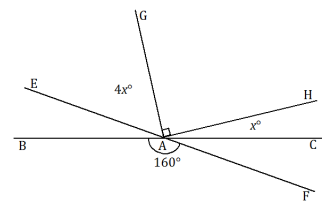What are the measures of ${\angle CAH }$ and ${\angle EAG}$

#### References

EngageNY Mathematics Grade 7 Mathematics > Module 6 > Topic A > Lesson 3Exit Ticket, Question #2

Grade 7 Mathematics > Module 6 > Topic A > Lesson 3 of the New York State Common Core Mathematics Curriculum from EngageNY and Great Minds. © 2015 Great Minds. Licensed by EngageNY of the New York State Education Department under the CC BY-NC-SA 3.0 US license. Accessed Dec. 2, 2016, 5:15 p.m..

Modified by The Match Foundation, Inc.

?